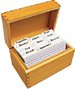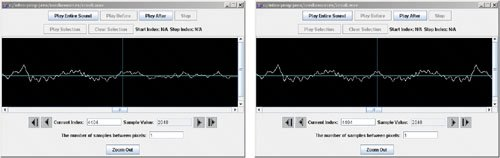# Section 9.5. Mirroring a Sound

[Page 306 (continued)]

### 9.5. Mirroring a Sound

Once we know how to play sounds forward and backward, mirroring the sound is the exact same process as mirroring pictures! Compare this to Program 19 (page 135). Do you see that this is the same algorithm, though we're dealing with a different medium?

##### Program 79. Mirror a Sound, Front to Back`/** * Method to mirror a sound front to back */ public void mirrorFrontToBack() { int length = this.getLength(); // save the length int mirrorPoint = length / 2; // mirror around this int value = 0; // hold the current value // loop from 0 to mirrorPoint for (int i = 0; i < mirrorPoint; i++) { value = this.getSampleValueAt(i); this.setSampleValueAt(length 1 i,value); } }`

To use this method try:

`> Sound s = new Sound(FileChooser.getMediaPath("croak.wav")); > s.explore(); > s.mirrorFrontToBack(); > s.explore();`

The length of the sound in the file "croak.wav" is 8,808, so the mirror point is at 4,404. Use the explorer to check the values on either side of the mirror point (Figure 9.5).

[Page 307]

##### Figure 9.5. Comparing the mirror point in the original sound (left) to the mirrored sound (right).Introduction to Computing & Programming Algebra in Java(c) A Multimedia Approach
ISBN: N/A
EAN: N/A
Year: 2007
Pages: 191